# What is Electrical Energy Definition Formula Unit of Electrical Energy

## What is Electrical Energy?

Before explaining what electrical energy is, let us try to review the potential difference between two points in an electric field.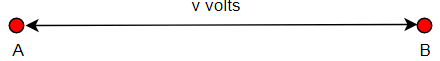Suppose potential difference between point A and point B in an electric is v volts.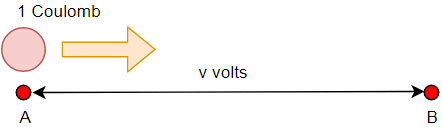As per the definition of potential difference we can say, if one positive unit electrical charge that is a body containing one-coulomb positive charge travels from point A to point B, it will do v joules work.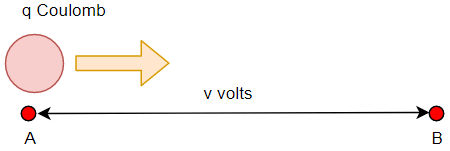Now instead of one-coulomb charge if q coulomb charge moves from point A to B, it will do vq joules work.

If the time taken by the q coulomb charge to travel from point A to B is t second, then we can write the rate of work done as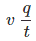Again, we define the work done per second as power. In that case, the termwould be electrical power. In differential form, we can write, electric power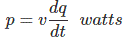Watt is the unit of power.

Now, if we place a conductor in between A and B, and through which the quantity of electric charge q coulomb is passing. The charge passing through a cross-section of the conductor per unit time (second) is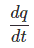It is nothing but the electric current i, through the conductor.
Now, we can write,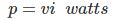If this current flows through the conductor for a time t, we can say the total work done by the charge is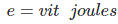We define this as electrical energy. So, we can say,

## Electrical Energy Definition

Electrical energy is the work done by electric charge. If current i ampere flows through a conductor or through any other conductive element of potential difference v volts across it, for time t second, the electric energy is,## Electrical Energy Formula

The expression of electric power isThe electrical energy is## Unit of Electrical Energy

Basically, we find the unit of electrical energy is joule. This equals to one watt X one second. Commercially, we also use other units of electrical energy, such as watt-hours, kilo watt hours, megawatt hours etc.

### Watt Hours

If one watt power is being consumed for 1 hour time, the energy consumed is one watt-hour.

### BOT Unit or Board of Trade Unit or Kwh

The practical, as well as a commercial unit of electrical energy, is kilowatt hour. The fundamental commercial unit is watt-hour and one kilowatt hour implies 1000 watt hours. The electrical supply companies take electric energy charges from their consumer per kilowatt hour unit basis. This kilowatt hour is board of trade unit that is BOT unit.

Want To Learn Faster? 🎓
Get electrical articles delivered to your inbox every week.
No credit card required—it’s 100% free.